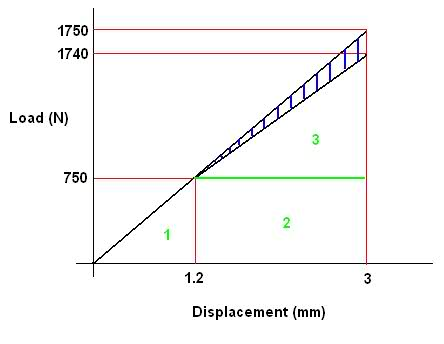# Area Calculations to find Jc energy Parmeter (simple maths)

## Homework Statement

I have been given the following load-displacement plots for the same specimin with two different crack lengths. The lines are identical up to a load od 750Ni need to calculate the area of the blue shaded region to obtain a value of Jc using a separate equation.

## Homework Equations

Area of triangle = 0.5(b)(h)
Area of rectangle = (b)(h)

## The Attempt at a Solution

I found the area of the largest triangle (tip at 1750)
Total Area = (0.5)(0.003)(1750)=2.625

Then split the lower half of the plot into 3 sections labeled 1,2&3. And calculated the areas
Area 1=(0.5)(0.0012)(750)=0.45
Area 2=(0.003-0.0012)(750)=1.35
Area 3=(0.5)(0.003-0.0012)(1740-750)=0.891

So Total Area - Area 1 -Area 2 - Area 3 = the blue shaded region
However i get a negative value,

Blue Shaded region = 2.625-0.45-1.35-0891 = -0.066

Just by looking at the picture, it shouldnt be a negative value, I cant see the problem in my method. Can any1 see what im doing wrong?

Any Help is appreciated
Thanks